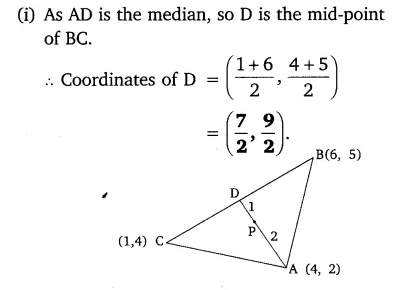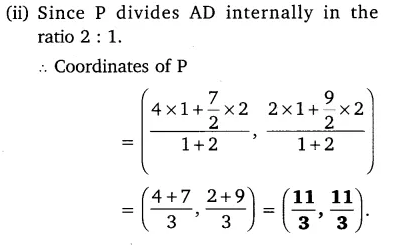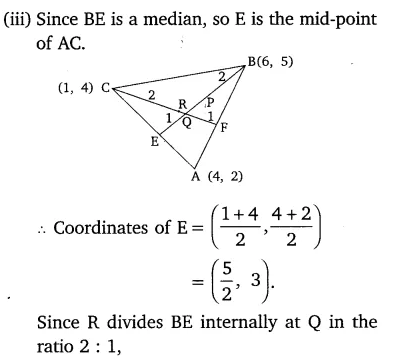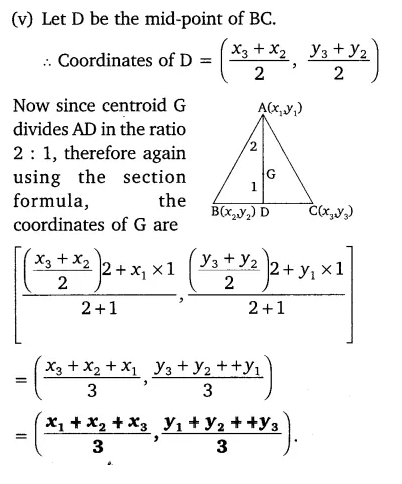# Let A(4, 2), B(6,5) and C(1, 4) be the vertices of ∆ABC

Let A(4, 2), B(6,5) and C(1, 4) be the vertices of ∆ABC.
(i) The median from A meters BC at D. Find the coordinates of the point D.
(ii) Find the coordinates of the point P on AD, such that AP: PD = 2:1.
(iii) Find the coordinates of points Q and R on medians BE and CF respectively, such that BQ: QE = 2: 1 and CR: RF = 2: 1.
(iv) What do you observe?
[ Note: The points which are common to all the three medians is called centroid and this point divides each median in the ratio 2: 1]
(v) If A(x1, y1), B(x2, y2) and C(x3, y3) are the vertices of ∆ABC, find the coordinates of the centroid of the triangles.